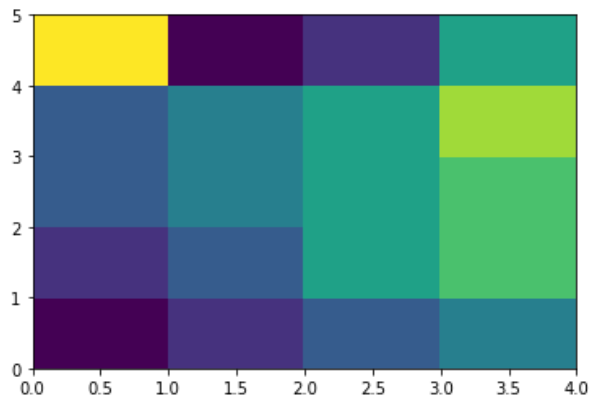# (27)Python热图

``````from pandas import DataFrame
import matplotlib.pyplot as plt

data=[{2,3,4,1},{6,3,5,2},{6,3,5,4},{3,7,5,4},{2,8,1,5}]
Index= ['I1', 'I2','I3','I4','I5']
Cols = ['C1', 'C2', 'C3','C4']
df = DataFrame(data, index=Index, columns=Cols)

plt.pcolor(df)
plt.show()
```Python```关注右侧公众号，随时随地查看教程
Python数据分析教程目录